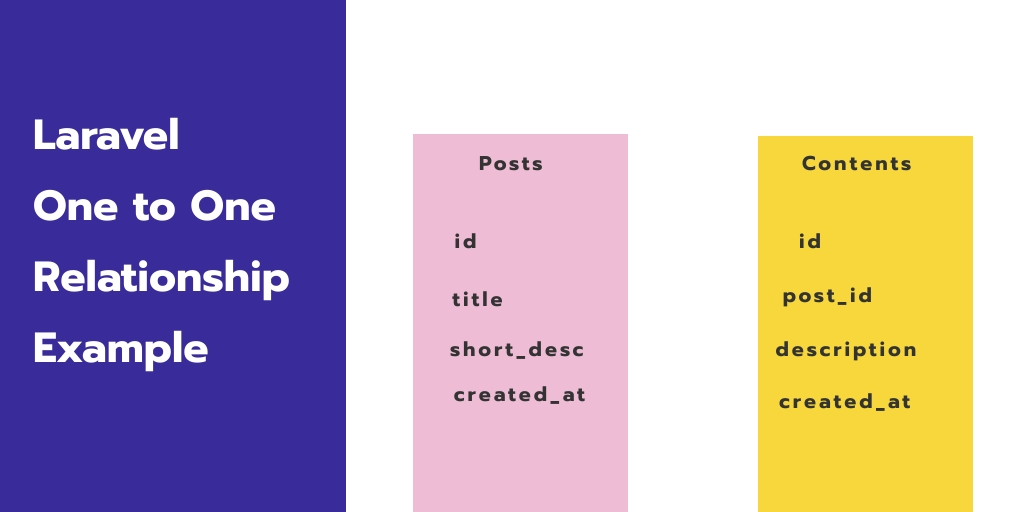Laravel 23-Dec-2020

### Laravel One to One Relationship Example

Laravel one to one relationship example tutorial. In this tutorial, you will learn about one to one relationships with examples.

A one-to-one relationship is a very basic relation. And using one to one relationship, you can insert, retrieve, update, and delete data with the eloquent model from the database table in laravel.

## Laravel Eloquent One to One Relationship Example

Let you have two tables name posts and contents. Using laravel migration, you can create both tables with the following fields:

Post table migration:

Schema::create('posts', function (Blueprint \$table) {

\$table->increments('id');

\$table->string('title');

\$table->text('short_desc');

\$table->timestamps();

});

Contents table migration:

Schema::create('contents', function (Blueprint \$table) {

\$table->increments('id');

\$table->integer('post_id')->unsigned();

\$table->text('description');

\$table->timestamps();

\$table->foreign('post_id')->references('id')->on('posts')

});

One to one relationship is one of the basic relationships. For example, a Post model would be associated with a Content model. To illustrate this relationship, we can create a post_content() method within the Post model and call the hasOne() method to relate the Content model.

<?php

namespace App;

use Illuminate\Database\Eloquent\Model;

class Post extends Model
{
public function post_content()
{
return \$this->hasOne('App\Content');
}
}

It is important to note that Eloquent establishes a foreign key based on the model name and should have a matching value id. Here post_id is the foreign_key of Content, So in order to create the relation.

#### The inverse of One to One Relationship Example

So far, we can access the content from the post. Let us now create an inverse relationship on the content model so that the post can access the model from it. To do this, we can use the belongsTo method for getting the post data on the content model.

<?php

namespace App;

use Illuminate\Database\Eloquent\Model;

class Content extends Model
{
public function post()
{
return \$this->belongsTo('App\Post');
}
}

We can now access the post content using the relation method like this:

`\$content = Post::find(10)->post_content;`

You can insert record from post and content table using one to one relationship

{
\$post = new Post;
\$post->title= "Hello world";
\$post->short_desc= "Hello world";
\$post->save();

\$content = new Content;
\$content->description= 'helllo world post description';
\$content->post_content()->save(\$content);
}

if you want to retrieve data from both post and content table, you can use one to one relationship as follow:

public function index()
{
// get post and content from Post Model
\$post = Post::find(1);
var_dump(\$post->title);
var_dump(\$post->post_content->description);

// get post data from PostContent model
\$post= Content::find(1)->post;
dd(\$post);

}

If you want to delete data from both tables posts and contents, you can use one to one relationship as follow:

public function delete()
{
\$post = Post::find(1);
\$post->delete();
}

## Conclusion

In this laravel one to one relationship example, you have learned how to implement one to one relationship in laravel. And as well as how to use this relationship.